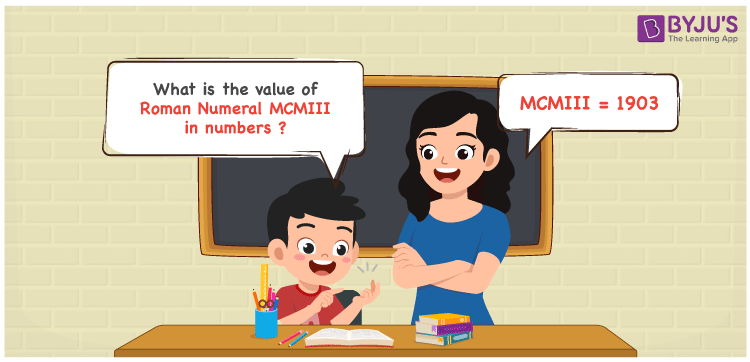Checkout JEE MAINS 2022 Question Paper Analysis : Checkout JEE MAINS 2022 Question Paper Analysis :

# MCMIII Roman Numerals

MCMIII Roman Numerals is 1903. Roman numerals are the system of expressing numbers using the Roman alphabet. Students can access the PDF of Roman Numerals 1 to 100 to learn the essential tricks of representing Roman letters from 1 to 100 natural numbers with ease. This also boosts confidence in solving complex problems effortlessly. Learn the simple method of writing the number form of Roman Numeral MCMIII in this article.

 Number Roman Numeral 1903 MCMIII

## How to Write MCMIII Roman Numerals?We can obtain the numerical value of MCMIII Roman Numerals by following the methods given below

First Method:

• Break the Roman Numerals into single letters
• MCMIII = M + (M – C) + I + I + I
• Write the numerical value of each letter and add/subtract them
• MCMIII = 1000 + (1000 – 100) + 1 + 1 + 1 = 1903

Second Method:

In this method, we consider the groups of Roman Numerals for addition or subtraction

• MCMIII = M + CM + III = 1000 + 900 + 3 = 1903
• Hence the numerical value of MCMIII Roman Numerals is 1903

## Video Lesson on Roman Numerals## Related Articles

Roman Numerals

Roman Numerals 1 to 50

Roman Numerals 1 to 1000

Roman Numerals Conversion

L Roman Numeral

Roman Numeral XXXIX

## Frequently Asked Questions on MCMIII Roman Numerals

### What is the number form of MCMIII Roman Numerals?

The number form of MCMIII Roman Numerals is 1903.

### What is the value of (500 – 455) + 1903 in Roman Numerals?

(500 – 455) + 1903 = 1948. The number 1948 in Roman Numerals is MCMXLVIII. Hence the value of (500 – 455) + 1903 in Roman Numerals is MCMXLVIII.

### Does MCMIII represent the number 1903?

Yes. The Roman Numeral MCMIII represents the number 1903.